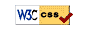# UM Math 216 On-Line Homework W20

## Hints and Tips

1. Start early, when each set opens.
2. Do homework0. It's easy & will help you with the homework system.
3. Don't submit partial answers. You have six submissions per problem.
4. Work the problems out by hand before entering them in the system. You can get a hardcopy of a problem set from the problm listing page of a problem set.
6. Use calculator syntax:
1. Carefully parenthesize. Type `cos(2pi)`, `e^(-ln(3))`, or `1/(1+x)`, not `cos 2pi`, `e^-ln3` or `1/1+x`. Note that `sin2x` is read as `sin(2)*x`, which is probably not what you want. Enter `sin(2x)` instead.
2. The square root is `sqrt(x)` or `x^(1/2)`.
3. Be accurate: the system demands four or five significant figures in your answers! Thus if the answer is pi, the answer 3.14159 will almost certainly be marked right, but the answer 3.14 will not.
7. Watch your case: `X` is different from `x`, `Sin` is different from `sin`, and `Pi` is different from `pi`. You want `pi`.

## Important InfoUM Math 216 On-Line HW